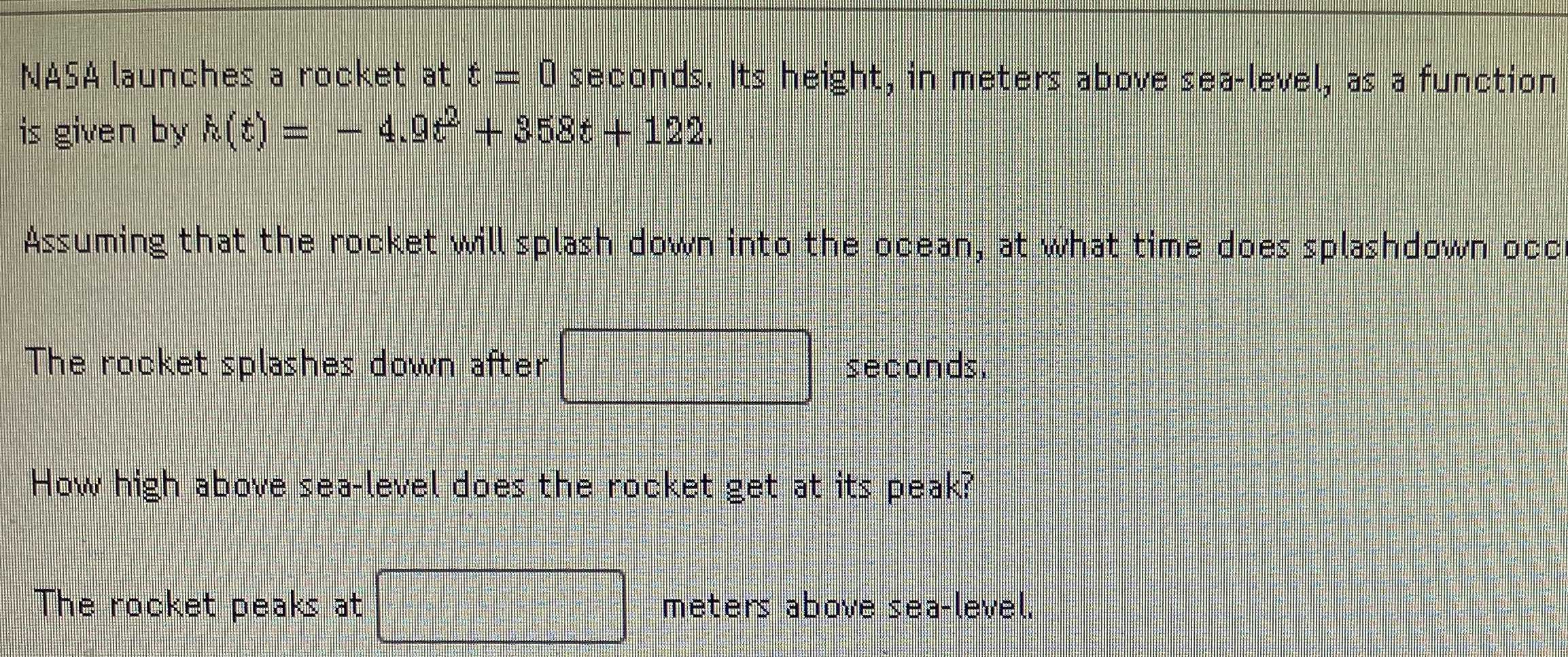### Still have math questions?

Algebra
QuestionNASA launches a rocket at $$t = 0$$ seconds, Its height, in meters above sea-level, as a function is given by $$h ( t ) = - 4.9 t ^ { 2 } + 358 t + 122$$ .

Assuming that the rocket will splash down into the ocean, at what time does splashdown occ

The rocket splashes down after _____ seconds,

How high above sea-level does the rocket get at its peak?

The rocket peaks at meters above sea-level,

$$h(t) = at^2+ bt+ c\\a= -4.9\\b= 358\\c= 122$$
2.    6660.98 meters,when t =$$- \frac{b}{2a}= \frac{358}{- 2\times(- 4.9)}$$